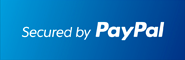﻿ #1 Statistics Solver for the TI-89 Calculator# #1 Statistics Solver for the TI-89 Calculator

## Statistics & Probability Made Easy### Works With TI92 Plus TI89 TI89 Titanium Voyage 200At the end of the PayPal checkout, you will be sent an email containing your key and software.Description

• Users have boosted their Statistics and Probability knowledge.
• Shows Step by Step solutions for most Statistics and Probability topics.
• Step by Step Confidence Intervals for mean, proportion, 2 means, 2 proportions, variance, slope, etc.
• Compute Sample Sizes for Confidence Intervals.
• Step by Step Tests of Significance (Z-Tests, T-Tests, ChiSquare Goodness of Fit and Independence tests, 2-sample T tests, 2 proportion Z tests, for Variance, etc)
• Step by Step Non-Parametric Tests: Wilcoxon Sign Test, Rank Sum Test, Signed Rank Test, Kruskal Wallis Test, Friedman Test, etc.
• Step by Step F Test for 2 variances
• Step by Step ANOVA to test multiple means.
• Step by Step 2-way ANOVA.
• Step by Step Linear Regression.
• Read about how Significant Tests: Type 1 Error, Type 2 Error, Power of a Test.
• Much easier handling than other Statistics packages: Choose to enter Data or Statistic values. Select Significant/Confidence Levels easily.
• Distributions: plot them, shade them where desired, compute probabilities, etc.
• Includes Binomial, Negative Binomial, Geometric, Normal, Student-t, Exponential, Poisson, Uniform, Sampling, Multinomial, Hypergeometric, F-, Chi-Square and more Distributions.
• Probability Checker for independent events, disjoint events. Also solves P(A or B)=P(A)+P(B)-P(A and B) for the unknown.
• Regressions: Linear , Quadratic, Cubic, Quartic, Sinusoidal, Logarithmic, Exponential, Power, MedMed
• Study Residuals and plot them
• Step by Step Derive the Coefficients for a Linear Regression Line, the Coefficient of Determination and the Correlation Coefficient.
• Read Theorems such as Central Limit Theorem, Random Variables, about Confidence Intervals and Tests, etc
• Also: Z-score Solver, Random Number Generator, etc
• 1 or 2 variable stats on mean, mode, median, standard deviation, variance, range, harmonic and geometric mean
• Plots: BoxPlots, x-y Plots, Scatter Plots, Histograms, Modified Box Plot